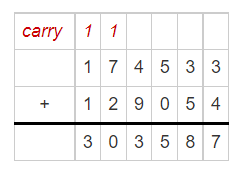Practice the questions of McGraw Hill Math Grade 4 Answer Key PDF Chapter 5 Test to secure good marks & knowledge in the exams.

Find each product using partial products. You can draw pictures for each problem on another sheet of paper.

Question 1.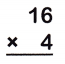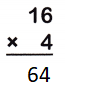Explanation:
The product of 16 and 4 is 64
take the carried value and place it in the column to the left.
So if you were multiplying numbers in the tens column and you needed to carry a number,
you would place that “number” in the hundreds column.

Question 2.Explanation:
The product of 58 and 6 is 348
take the carried value and place it in the column to the left.
So if you were multiplying numbers in the tens column and you needed to carry a number,
you would place that “number” in the hundreds column.

Question 3.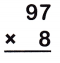Explanation:
The product of  97 and 8 is 776
take the carried value and place it in the column to the left.
So if you were multiplying numbers in the tens column and you needed to carry a number,
you would place that “number” in the hundreds column.

Question 4.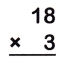Explanation:
The product of  18 and 3 is 54
take the carried value and place it in the column to the left.
So if you were multiplying numbers in the tens column and you needed to carry a number,
you would place that “number” in the hundreds column.

Question 5.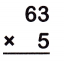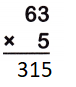Explanation:
The product of  63 and 5 is 315
take the carried value and place it in the column to the left.
So if you were multiplying numbers in the tens column and you needed to carry a number,
you would place that “number” in the hundreds column.

Question 6.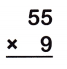Explanation:
The product of  55 and 9 is 495
take the carried value and place it in the column to the left.
So if you were multiplying numbers in the tens column and you needed to carry a number,
you would place that “number” in the hundreds column.

Find each product.

Question 7.Explanation:
The product of  266 and 6 is 1596
take the carried value and place it in the column to the left.
So if you were multiplying numbers in the tens column and you needed to carry a number,
you would place that “number” in the hundreds column.

Question 8.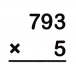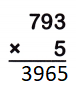Explanation:
The product of  793 and 5 is 3965
take the carried value and place it in the column to the left.
So if you were multiplying numbers in the tens column and you needed to carry a number,
you would place that “number” in the hundreds column.

Question 9.Explanation:
The product of  625 and 8 is 5000
take the carried value and place it in the column to the left.
So if you were multiplying numbers in the tens column and you needed to carry a number,
you would place that “number” in the hundreds column.

Question 10.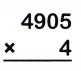Explanation:
The product of  4905 and 4 is 19620
take the carried value and place it in the column to the left.
So if you were multiplying numbers in the tens column and you needed to carry a number,
you would place that “number” in the hundreds column.

Question 11.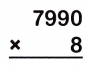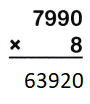Explanation:
The product of 7990 and 8 is 63920
take the carried value and place it in the column to the left.
So if you were multiplying numbers in the tens column and you needed to carry a number,
you would place that “number” in the hundreds column.

Question 12.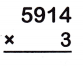Explanation:
The product of  5914 and 3 is 17742
take the carried value and place it in the column to the left.
So if you were multiplying numbers in the tens column and you needed to carry a number,
you would place that “number” in the hundreds column.

Question 13.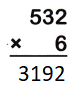Explanation:
The product of  532 and 6 is 3192
take the carried value and place it in the column to the left.
So if you were multiplying numbers in the tens column and you needed to carry a number,
you would place that “number” in the hundreds column.

Question 14.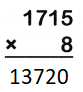Explanation:
The product of  1715 and 8 is 13720
take the carried value and place it in the column to the left.
So if you were multiplying numbers in the tens column and you needed to carry a number,
you would place that “number” in the hundreds column.

Question 15.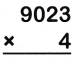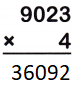Explanation:
The product of  9023 and 4 is 36092
take the carried value and place it in the column to the left.
So if you were multiplying numbers in the tens column and you needed to carry a number,
you would place that “number” in the hundreds column.

Find each product. You can draw arrays for each problem on another sheet of paper.

Question 16.
42 × 25 = ____Explanation:
The product of 42 x 25 = 1050
take the carried value and place it in the column to the left.
So if you were multiplying numbers in the tens column and you needed to carry a number,
you would place that “number” in the hundreds column.

Question 17.
13 × 29 = ___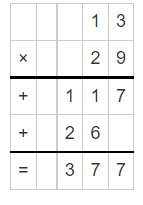Explanation:
The product of 13 and 29 is 377

Question 18.
71 × 33 = ___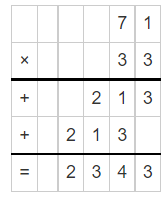Explanation:
The product of 71 and 33 is 2343

Question 19.
17 × 31 = ___Explanation:
The product of 17 and 31 is 527

Question 20.
24 × 53 = ___Explanation:
The product of 24 and 53 is 1272

Question 21.
49 × 14 = ___Explanation:
The product of 49 and 14 is 686

Find each product using mental math.

Question 22.
400 × 50 = ___
20,000
Explanation:
4 x 5 = 20

Question 23.
30 × 600 = ___
18,000
Explanation:
3 x 6 = 18

Question 24.
70 × 400 = ____
28,000
Explanation:
7 x 4 = 28

Use rounding or compatible numbers to estimate each product.

Question 25.
52 × 61 = ___
3000
Explanation:
52 is rounded to 50
and 61 is rounded to 60
5 x 6 = 30

Question 26.
47 × 11 = ___
500
Explanation:
47 is rounded to 50
11 is rounded to 10
5 x 1 = 5

Question 27.
897 × 9 = ___
8100
Explanation:
897 is rounded to 900
9 x 9 = 81

Solve. Make an estimate to show that each answer is reasonable.

Question 28.
A hotel has 239 beds. There are 4 blankets for each bed. How many blankets does the hotel have?
_____________________
The hotel staff washes the blankets. So far, they have washed 402 blankets. How many blankets are left to wash?
_____________________
956 blankets in total
554 blankets are left to wash
Explanation:
hotel has 239 beds.
and  4 blankets for each bed.
239 x 4 = 956 blankets
they have washed 402 blankets.
956 – 402 = 554
so, 554 blankets are left to wash

Question 29.
A used car lot has 16 rows of cars to sell. There are 12 cars in each row. How many cars are for sale in the used car lot?Explanation:
16 rows of cars to sell.
There are 12 cars in each row.
16 x 12 = 192
192 cars are for sale in the used car lot

Question 30.
Marco has 31 green marbles, 19 red marbles, and 22 blue marbles. How many marbles does he have in all?
_________________
Nicole has 209 marbles. How many more marbles does Nicole have than Marco?
_________________
Marco has 72 marbles
137 more marbles that Nicole have than Marco
Explanation:
31 green marbles, 19 red marbles, and 22 blue marbles.
31 + 19 + 22 = 72 marbles
Nicole has 209 marbles.
209 – 72 = 137

Question 31.
One city has a population of 174,533. A nearby city has 129,054 people. How many people live in the two communities.